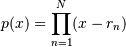# numpy.polynomial.polynomial.polyvalfromroots¶

`numpy.polynomial.polynomial.``polyvalfromroots`(x, r, tensor=True)[source]

Evaluate a polynomial specified by its roots at points x.

If r is of length N, this function returns the valueThe parameter x is converted to an array only if it is a tuple or a list, otherwise it is treated as a scalar. In either case, either x or its elements must support multiplication and addition both with themselves and with the elements of r.

If r is a 1-D array, then p(x) will have the same shape as x. If r is multidimensional, then the shape of the result depends on the value of tensor. If tensor is ``True` the shape will be r.shape[1:] + x.shape; that is, each polynomial is evaluated at every value of x. If tensor is `False`, the shape will be r.shape[1:]; that is, each polynomial is evaluated only for the corresponding broadcast value of x. Note that scalars have shape (,).

New in version 1.12.

Parameters: x : array_like, compatible object If x is a list or tuple, it is converted to an ndarray, otherwise it is left unchanged and treated as a scalar. In either case, x or its elements must support addition and multiplication with with themselves and with the elements of r. r : array_like Array of roots. If r is multidimensional the first index is the root index, while the remaining indices enumerate multiple polynomials. For instance, in the two dimensional case the roots of each polynomial may be thought of as stored in the columns of r. tensor : boolean, optional If True, the shape of the roots array is extended with ones on the right, one for each dimension of x. Scalars have dimension 0 for this action. The result is that every column of coefficients in r is evaluated for every element of x. If False, x is broadcast over the columns of r for the evaluation. This keyword is useful when r is multidimensional. The default value is True. values : ndarray, compatible object The shape of the returned array is described above.

Examples

```>>> from numpy.polynomial.polynomial import polyvalfromroots
>>> polyvalfromroots(1, [1,2,3])
0.0
>>> a = np.arange(4).reshape(2,2)
>>> a
array([[0, 1],
[2, 3]])
>>> polyvalfromroots(a, [-1, 0, 1])
array([[ -0.,   0.],
[  6.,  24.]])
>>> r = np.arange(-2, 2).reshape(2,2) # multidimensional coefficients
>>> r # each column of r defines one polynomial
array([[-2, -1],
[ 0,  1]])
>>> b = [-2, 1]
>>> polyvalfromroots(b, r, tensor=True)
array([[-0.,  3.],
[ 3., 0.]])
>>> polyvalfromroots(b, r, tensor=False)
array([-0.,  0.])
```

#### Previous topic

numpy.polynomial.polynomial.polyfromroots

#### Next topic

numpy.polynomial.polynomial.polyfit